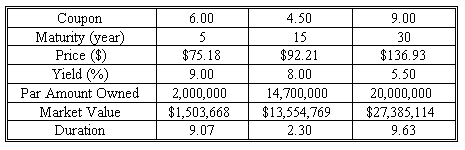### CFA Practice Question

There are 266 practice questions for this study session.

### CFA Practice Question

Darlene Mainee owns a portfolio that consists of three bonds. What is the duration of the bond portfolio?A. 6.6862
B. 7.2661
C. 7.0552
Explanation: This calculation is very similar to a Weighted Average Cost of Capital computation. The first step requires that each bond be assessed for its relative weight in the portfolio. The corresponding weight is then multiplied by the bond's duration, which provides the bond's contribution to the overall portfolio duration.

Note: While this problem provides the bond's market value (already computed), this could be left to candidates to determine on an exam. The bond's market value is simply the bond's price (relative to par) multiplied by the corresponding par value.

The detailed computation follows:
Total Market Value of Portfolio = Market value of each bond added together = \$1,503,668 + \$13,554,769 + \$27,385,114 =\$42,443,551

Next, compute the relative weight of each bond in the portfolio.
Weight of 6.00%, 2,000,000 par value bond in portfolio (duration of 9.07) = Market value of Bond /Market Value of Total Portfolio = 0.035
Weight of 4.50%, 14,700,000 par value bond in portfolio (duration of 2.30)= Market value of Bond /Market Value of Total Portfolio = 0.319
Weight of 9.00%, 20,000,000 par value bond in portfolio (duration of 9.63) = Market value of Bond /Market Value of Total Portfolio = 0.645

The portfolio's duration equals the weighted average of each bond's duration multiplied by its weight:

0.035 * 9.07 + 0.319 * 2.30 + 0.645 * 9.63
= 0.3212 + 0.7332 + 6.2117
= 7.2661